# GSEB Solutions Class 11 Maths Chapter 11 Conic Sections Miscellaneous Exercise

Gujarat Board GSEB Textbook Solutions Class 11 Maths Chapter 11 Conic Sections Miscellaneous Exercise Textbook Questions and Answers.

## Gujarat Board Textbook Solutions Class 11 Maths Chapter 11 Conic Sections Miscellaneous Exercise

Question 1.
If a parabolic reflector is 20 cm in diameter and 5 cm deep, find the focus.
Solution:
Taking vertex of the parabolic reflector at origin, x-axis along the axis of parabola, the equation of parabola is y2 = 4ax.
Given depth 5 cm, diameter 20 cm.
∴ (5, 10) point lies on parabola.
(10)2 = 4a(5)
∴ a = 5.
∴ Focus is (a, 0), i.e., (5, 0), which is the mid-point of the given diameter.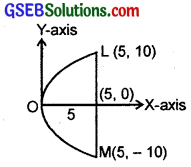Question 2.
An arch is in the form olf a parabola with its axis vertical. The arch is 10 m high and 5 m wide at the base. How wide is it 2 m from the vertex of the parabola?
Solution:
∵ Axis of the parabola is vertical.
∴Its equation is x2 = 4ay
Arch is 10 m high and 5 m wide at base.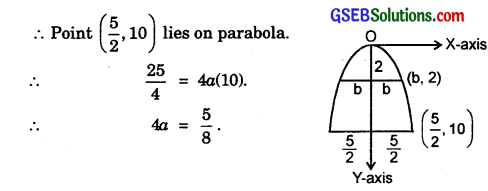∴ Equation of parabola becomes x2 = $$\frac{5}{8}$$y.
Let width of arch 2 m from vertex is 2b, then, point (b, 2) lies on parabola.
b2 = $$\frac{5}{8}$$.2 = $$\frac{5}{4}$$
∴ b = $$\frac{\sqrt{5}}{2}$$.
∴ Width of arch is 2b = 2.$$\frac{\sqrt{5}}{2}$$m = $$\sqrt{5}$$m = 2.23 m (approx.).

Question 3.
The cable of a uniformly loaded suspension bridge hangs in the form of a parabola.
The roadway, which is horizontal and 100 m long, is supported by vertical wires attached to the cable, the longest wire being 30 m and the shortest being 6 m.
Find the length of a supporting wire attached to the roadway 18 m from the middle.
Solution:
The cable is in the form of a parabola x2 = 4ay.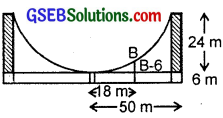Focus is at the middle of the cable and, shortest and longest vertical supports are 6 m and 30 m, and roadways is 100 m long.
∴ Point (50, 24) lies on the parabola.
∴ (50)2 = 4a(2a) or 4a = $$\frac{625}{6}$$.
∴ Equation of parabola is x2 = $$\frac{625}{6}$$y.
Let the support at 18 m from middle be B m. Then, (18, B – 6) lies on the parabola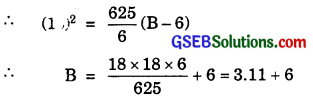= 9.11 (approx.)
∴ Length of support is 9.11 m (approx.)Question 4.
An arch is in the form of a semi-ellipse. It is 8 m wide and 2 m high at the centre. Find the height of the arch at a point 1.5 m from one end.
Solution:
Since the arch is 8 m wide and 2 m high at centre in the form of a semi-ellipse, so
arch is a part of ellipse whose semi-major and semi-minor axes are 4 m and 2 m respectively.
i.e., a = 4, b = 2.
∴ Its equation is $$\frac{x^{2}}{16}$$ + $$\frac{y^{2}}{4}$$ = 1
or x2 + 4y2 = 16.
A point 1.5 m from one end of arch is (4 – 1.5) m = 2.5 m from origin.
Height of the arch at this point is given by value of it obtained by putting x = 2.5.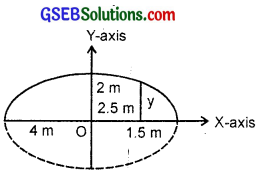∴ (2.5)2 + 4y2 = 16.
⇒ 4y2 = 16 – 6.25 = 9.75
⇒ y = $$\frac{\sqrt{9.75}}{2}$$ = $$\frac{3.12}{2}$$ = 1.56 (approx.)
∴ Height of the arch is 1.56 m (approx.)Question 5.
A rod of length 12 cm moves with its ends always touching the co-ordinate axes.
Determine the equation of the locus of a point P on the rod, which is 3 cm from the end in contact with the x-axis.
Solution:
Let AB = 12 cm is the rod and P(x, y) is point on the rod such that
PA = 3 cm.
∴ PB = 9 cm.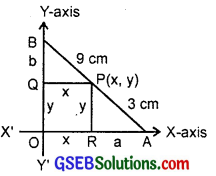Draw PQ ⊥ OB and PR ⊥ OA.
Let BQ = b and AR = a.
Then, from similar ∆s BQP and PRA, we have:
$$\frac{b}{9}$$ = $$\frac{y}{3}$$ and $$\frac{a}{3}$$ = $$\frac{x}{9}$$.
⇒ b = 3y and a = $$\frac{1}{3}$$x.
∴ OA = x + a = x + $$\frac{1}{3}$$x = $$\frac{4}{3}$$x
and OB = y + b = y + 3y = 4y.
Using OA2 + OB2 = AB2, we have: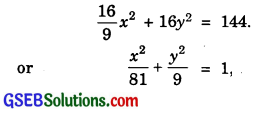which is locus of P.Question 6.
Find the area of the triangle formed by the lines joining the vertex of the parabola x2 = 12y to the ends of its latus rectum.
Solution:
Parabola is x2 = 12y.
∴ Length of latus rectum AB is 4a = 12.
Also ⊥ distance p = ON of vertex O from latus rectum AB is given by
(6)2 = 12p or p = 3.
∴ Area of ∆ formed by joining vertex
O the ends A and B of latus rectum is the area of ∆ OAB
= $$\frac{1}{2}$$ × 12 × 3 = 18 sq. units.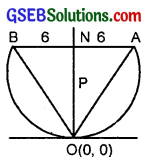Question 7.
A man running a race-course, notes that the sum of the distances from the two flag posts from him is always 10 m and the distance between the flag post is 8 m.
Find the equation of the path traced by the man.
Solution:
The path traced by the man according to the given condition will be an ellipse,
whose foci S and S’ will be the flag posts and the sum of distances of man P from S and S’ is equal to major axis.
If $$\frac{x^{2}}{a^{2}}$$ + $$\frac{y^{2}}{b^{2}}$$ = 1 is eqn. of the ellipse,
then, PS + PS’ = 2a = 10.
Now, since a = 5 m so
SS’ = 2ae = 8.Question 8.
An equilateral triangle is inscribed in the parabola y2 = 4ax, where one vertex is at the vertex of the parabola. Find the length of the side of the triangle.
Solution:
Equation of parabola is y2 = 4ax.
Let p is the side of the equilateral AOAB whose one vertex is at the vertex of parabola.
Then, by symmetry AB is ⊥ to the axis ON of parabola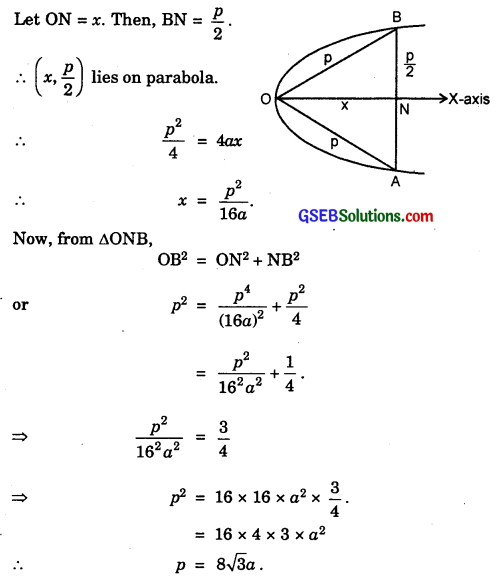∴ Side of the traiangle is 8$$\sqrt{3}$$a.